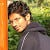# Problem statement

`Input: numRows = 5Output: [ , [1, 1], [1, 2, 1], [1, 3, 3, 1]…`

`…`

# Problem statement

`Input: head = [1, 2, 3, 4] Output: [2, 1…`

# Problem statement

`Input: l1 = [1, 2, 4], l2 = [1, 3, 4]Output: [1, 1, 2, 3, 4…`

# Problem statement

`Input: nums1 = [1, 3], nums2 = Output: 2.00000 …`

# Problem statement

`Input: n = 3Output: ["((()))", "(()())", "(())()", "()(())", "()()()"]`
`Input: n = 1Output: ["()"]`
`- 1 <= n <= 8`

# Explanation

## Brute force